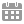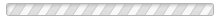# 宇晴醫療中心 - 科進語言及學習方案 病人資料

1.2.

3.

4.

• *
I. 病人基本资料
5.6.

7.

• *
• *
• *
• *
8.

9.

• *
• *
• *
10.

II. 病人母亲怀孕期资料
11.

12.

13.

• *
14.

15.

16.

17.

• *
18.

• *
19.

• *
20.

• *
21.

22.

• *
23.

• *
III.病人母亲分娩过程资料
24.

• *
25.

• *
26.

• *
27.

• *
28.

• *
29.

• *
30.

• *
31.

• *
32.

• *
33.

A.母亲基本数据
34.

*
35.36.

• *
• *
37.

• *
• *
38.

39.

• *
• *
B.父亲基本资料
40.

41.42.

• *
• *
43.

• *
• *
44.

45.

• *
• *
46.

47.

48.

• *
49.

• *
50.

51.

• *
52.

53.

• *
54.

• *
55.

56.

• *
57.

58.

• *
59.

• *
60.

61.

• *
62.

63.

• *
VI. 语言背景

64.

• *
65.

• *
66.

• *
67.

68.

• *

69.

• *
70.

• *
71.

• *
72.

73.

• *

74.

• *
75.

• *
76.

• *
77.

78.

• *

79.

• *
80.

• *
81.

• *
82.

83.

• *
VII. 其他资料
84.

85.

86.

• *
87.

• *
88.

• *
89.

• *
90.

• *
91.

• *
92.

• *
93.

94.

• *
95.

Q-CHAT-10 (幼儿自闭症量化检查表)

https://www.wjx.cn/jq/35228475.aspx

AQ-10 (儿童版) - 自闭症光谱量表

https://www.wjx.cn/jq/35228850.aspx问卷星 提供技术支持